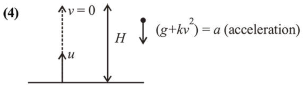# A small ball of mass $m$ is thrown upward with velocity u from the ground.

Question:

A small ball of mass $m$ is thrown upward with velocity $u$ from the ground. The ball experiences a resistive force $m k v^{2}$ where $v$ is its speed. The maximum height attained by the ball is :

1. (1) $\frac{1}{2 k} \tan ^{-1} \frac{k u^{2}}{g}$

2. (2) $\frac{1}{k} \ln \left(1+\frac{k u^{2}}{2 g}\right)$

3. (3) $\frac{1}{k} \tan ^{-1} \frac{k u^{2}}{2 g}$

4. (4) $\frac{1}{2 k} \ln \left(1+\frac{k u^{2}}{g}\right)$

Correct Option: , 4

Solution:$\vec{F}=m k v^{2}-m g$           $\left(\because m g\right.$ and $m k v^{2}$ act opposite to each other)

$\vec{a}=\frac{\vec{F}}{m}=-\left[k v^{2}+g\right]$

$\Rightarrow v \cdot \frac{d v}{d h}=-\left[k v^{2}+g\right]$              $\left(\because a=v \frac{d v}{d h}\right)$

$\Rightarrow \int_{u}^{0} \frac{v \cdot d v}{k v^{2}+g}=\int_{0}^{h} d h$

$\Rightarrow \frac{1}{2 k} \ln \left[k v^{2}+g\right]_{u}^{0}=-h$

$\Rightarrow \frac{1}{2 k} \ln \left[\frac{k u^{2}+g}{g}\right]=h$